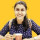1. Home >
2. Apps >
3. Groups >

# GATE Electronics & Communication Engineering Sample Questions - IV

Question asked by Ankita Katdare in #Electronics on Jul 8, 2011Ankita Katdare · Jul 8, 2011
Rank A1 - PRO
Q1 A circuit has a resistance of 11 W, a coil of inductive reactance 120 W, and a capacitor with a 120-W reactance, all connected in series with a 110-V, 60-Hz power source. What is the potential difference across each circuit element?

A) (a) VR = 110 V, (b) VL = VC = 1.2 Kv
B) (a) VR = 120 V, (b) VL = VC = 2.4 kV
C) (a) VR = 4.8 V, (b) VL = VC = 0 kV
D) (a) VR = 5.0 V, (b) VL = VC = 8.0 V

Q-2 Applying DeMorgan's theorem to the expression , we get

A) (A+B)+C
B) A(B + C)
C) Both A & B
D) None of above

Q-3
Express the decimal number 57 in binary.
A) 100101
B) 111010
C) 110010
D) 111001

Q-4 A vertical electric dipole antenna

a) radiates uniformly in all directions.
b) radiates uniformly in all horizontal directions, but more strongly in the vertical direction.
c) radiates most strongly and uniformly in the horizontal directions
d) does not radiate in the horizontal directions

Q-5
A particle oscillates according to the equation y=5.0 cos 23 t, where y is in centimeters. Find its frequency of oscillation and its position at t=0.15 s.

a) f = 23 Hz, y = -4.8 cm
B) f = 3.7 Hz, y = -5.0 cm
C) f = 3.7 Hz, y = -4.8 cm
D) f = 3.7 Hz, y = +4.8 cm

Q-6 A 10.0-µF capacitor is in series with a 40.0-W resistance, and the combination is connected to a 110-V, 60.0-Hz line. Calculate (a) the capacitive reactance, (b) the impedance of the circuit, (c) the current in the circuit, (d) the phase angle between current and supply voltage

A) (a) 0.0038W (b) 305W (c) 0.415 A (d) voltage lags by 8.58°
B) (a) 266W (b) 269W (c) 0.409 A (d) voltage lags by 81.4°
C) (a) 16 kW (b) 72 kW (c) 2.75 A (d) voltage lags by 6.63°
D) (a) 2.6 kW (b) 262W (c) 0.256 MA (d) voltage leads by 81.4°

Q-7 A circuit has a resistance of 11 W, a coil of inductive reactance 120 W, and a capacitor with a 120-W reactance, all connected in series with a 110-V, 60-Hz power source. What is the potential difference across each circuit element?

A) (a) VR = 110 V, (b) VL = VC = 1.2 Kv
B) (a) VR = 120 V, (b) VL = VC = 2.4 kV
C) (a) VR = 4.8 V, (b) VL = VC = 0 kV
D) (a) VR = 5.0 V, (b) VL = VC = 8.0 V

Q-8
What is the primary function of multiplexing?

A ) To match the frequency range of a signal to a particular channel.
B ) To reduce the bandwidth of a signal.
C ) To select one radio channel from a wide range of transmitted channels.
D ) To allow a number of signals to make use of a single communications channel.

Q-9
A second step to further increase system capacity is a digital access method called TDMA (Time Division Multiple Access). Using the same frequency channelization and reuse as FDMA analog but adding a time sharing element, the effective capacity is:

A) Doubled
B) Tripled
C) Reduced by one third
D) Unchanged

Q-10
What are Pseudo-Random noise sequences, or P/N Sequences?

A) P/N Sequences are known sequences which exhibit the properties or chracteristics of random sequences
B) P/N Sequences can be used to logically isolate users on the same physical (frequency) channel
C) P/N Sequences appear as random noise to everyone else, except to the transmitter and intended receiver
D) All of the above

Q-11 An op-amp integrator has a square-wave input. The output should be

A ) a sine wave.
B) a triangle wave
C) a square wave.
D) pure DC.

Q-12 What is the relationship between the series and parallel resonant frequencies of a quartz crystal?

A) They are equal.
B) Parallel resonant frequency is approximately 1 kHz higher than series resonant frequency
C) Series resonant frequency is approximately 1 kHz higher than parallel resonant frequency.
D) none of the above

Q-13 Which FET amplifier(s) has (have) a phase inversion between input and output signals?
A) common-gate
B) common-drain
C) common-source
D) all of the above

TRY TO FIND ANSWER TO ALL THE ABOVE QUESTIONS AND WRITE THEM IN REPLIES BELOW THIS. Posted in: #Electronics

## You must log-in or sign-up to reply to this post.

Click to Log-In or Sign-Up